# Addition of 3 or More Single Digit Numbers

Advertisements

Add the single digit numbers.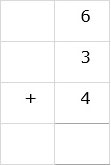### Solution

Adding three numbers involves two steps

Step 1:

We add the first two numbers; 6 + 3 = 9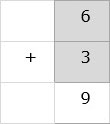Step 2:

Next we add the sum to third number; 9 + 4 = 13

We write down the result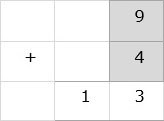So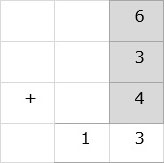Add the numbers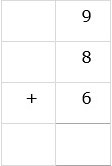### Solution

Step 1:

We add the three numbers by counting forward here. We start counting from the largest number 9 and the count forward 8 to get 17Step 2:

and from 17, we further count forward 6 to get 23, which is the sum.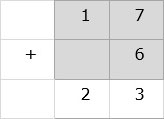So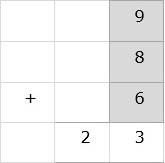Advertisements